Multiplication of matrices

Chapter 3 Class 12 Matrices
Concept wise

Here, scalar means a number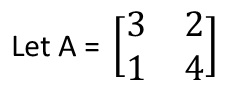What will be 3A?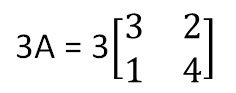Here, 3 will be multiplied to each element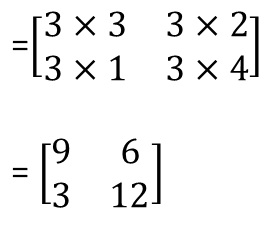Let’s take another example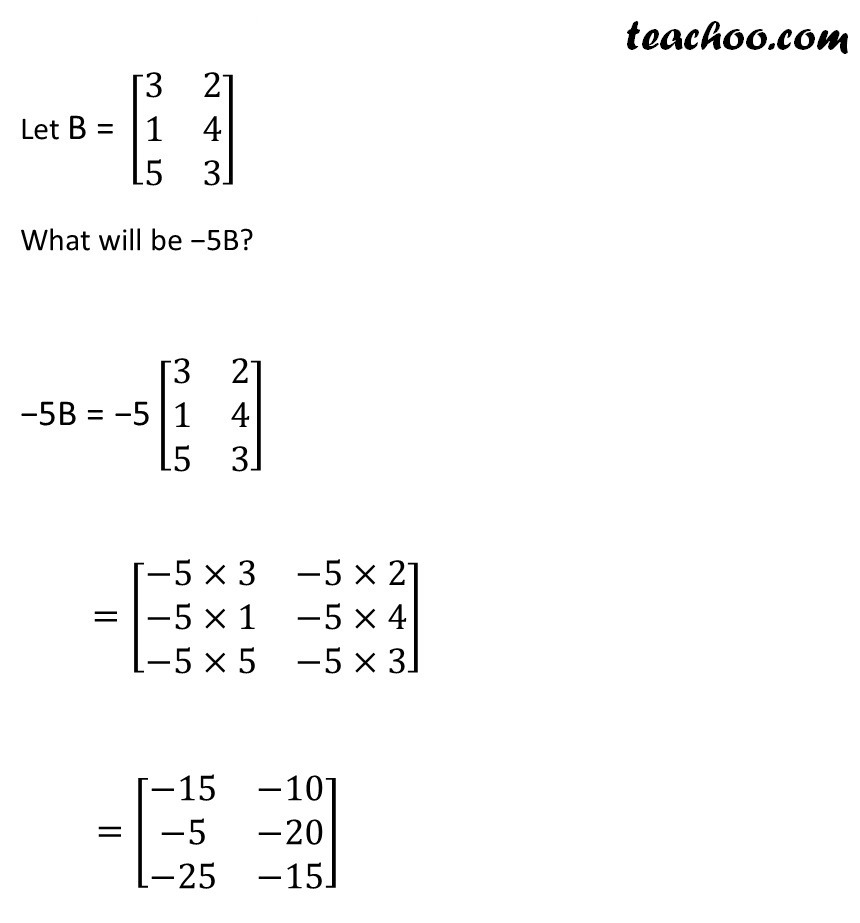Note: Multiplying a number to a matrix is different than

## What about negative of a matrix?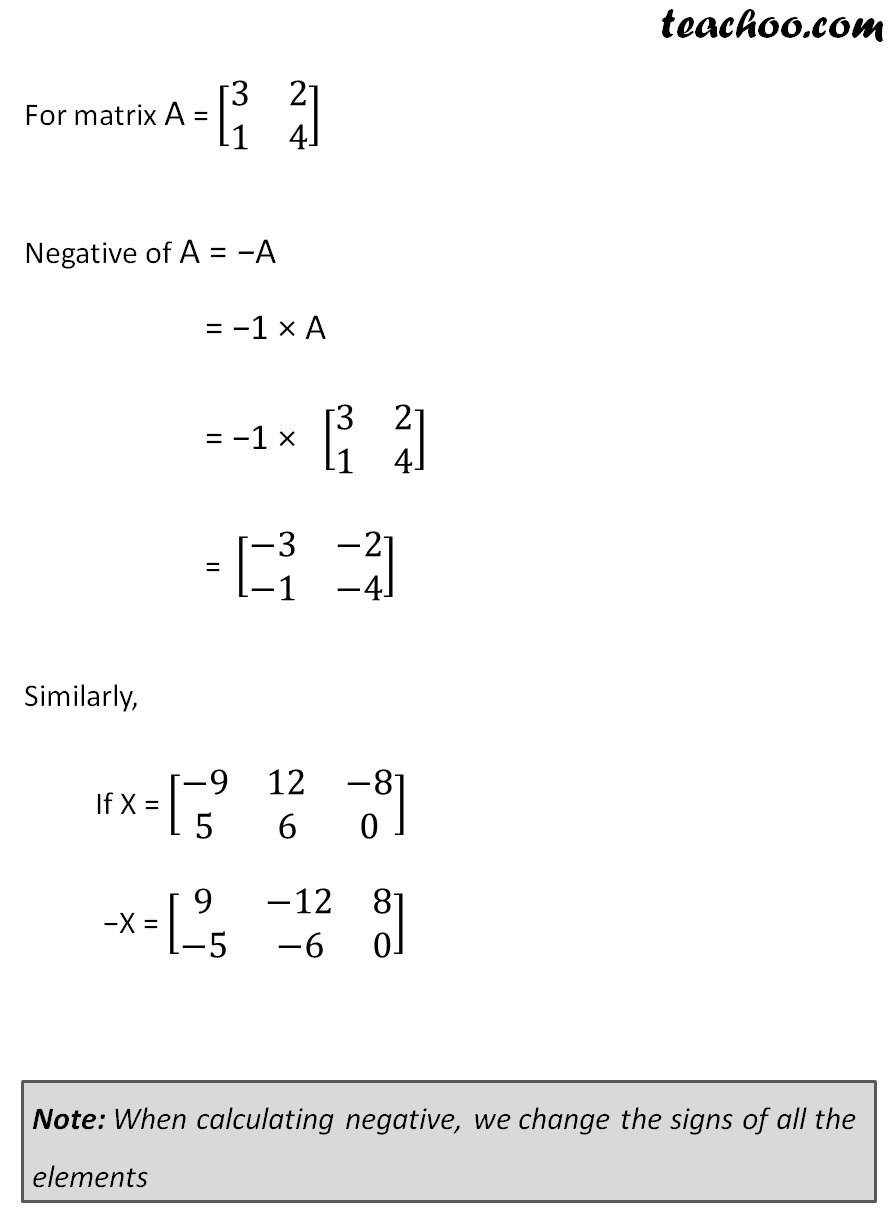Get live Maths 1-on-1 Classs - Class 6 to 12

### Transcript

Let A = [■8(3&[email protected]&4)] 3A = 3[■8(3&[email protected]&4)] =[■8(3×3&3×[email protected]×1&3×4)] = [■8(9&[email protected]&12)] Let B = [■8(3&[email protected]&[email protected]&3)] −5B = −5[■8(3&[email protected]&[email protected]&3)] =[■8(−5×3&−5×[email protected]−5×1&−5×[email protected]−5×5&−5×3)] =[■8(−15&−[email protected]−5&−[email protected]−25&−15)] For matrix A = [■8(3&[email protected]&4)] Negative of A = −A = −1 × A = −1 × [■8(3&[email protected]&4)] = [■8(−3&−[email protected]−1&−4)] Similarly, If X = [■8(−9&12&−[email protected]&6&0)] −X = [■8(9&−12&[email protected]−5&−6&0)] Note: When calculating negative, we change the signs of all the elements